Courses

# Test: Symmetry - 2

## 20 Questions MCQ Test Mathematics (Maths) Class 6 | Test: Symmetry - 2

Description
This mock test of Test: Symmetry - 2 for Class 6 helps you for every Class 6 entrance exam. This contains 20 Multiple Choice Questions for Class 6 Test: Symmetry - 2 (mcq) to study with solutions a complete question bank. The solved questions answers in this Test: Symmetry - 2 quiz give you a good mix of easy questions and tough questions. Class 6 students definitely take this Test: Symmetry - 2 exercise for a better result in the exam. You can find other Test: Symmetry - 2 extra questions, long questions & short questions for Class 6 on EduRev as well by searching above.
QUESTION: 1

Solution:
QUESTION: 2

Solution:
QUESTION: 3

### Find the number of lines of symmetry of the following figure: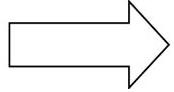Solution:
QUESTION: 4

Find the number of lines of symmetry of the following figure: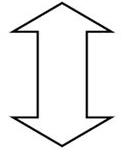Solution:
QUESTION: 5

Find the number of lines of symmetry in regular hexagon.

Solution:
QUESTION: 6

Letter ‘A’ of the English alphabet have reflectional symmetry (i.e., symmetry related to mirror reflection) about.

Solution:
QUESTION: 7

Letter ‘C’ of the English alphabet have reflectional symmetry (i.e., symmetry related to mirror reflection) about.

Solution:
QUESTION: 8

Letter ‘E’ of the English alphabet have reflectional symmetry (i.e., symmetry related to mirror reflection) about.

Solution:
QUESTION: 9

Letter ‘H’ of the English alphabet have reflectional symmetry (i.e., symmetry related to mirror reflection) about.

Solution:
QUESTION: 10

Letter ‘M’ of the English alphabet have reflectional symmetry (i.e., symmetry related to mirror reflection) about.

Solution:
QUESTION: 11

Letter ‘T’ of the English alphabet have reflectional symmetry (i.e., symmetry related to mirror reflection) about.

Solution:
QUESTION: 12

Letter ‘G’ of the English alphabet have reflectional symmetry (i.e., symmetry related to mirror reflection) about.

Solution:
QUESTION: 13

Letter ‘D’ of the English alphabet have reflectional symmetry (i.e., symmetry related to mirror reflection) about.

Solution:
QUESTION: 14

Letter ‘B’ of the English alphabet have reflectional symmetry (i.e., symmetry related to mirror reflection) about.

Solution:
QUESTION: 15

Letter ‘I’ of the English alphabet have reflectional symmetry (i.e., symmetry related to mirror reflection) about.

Solution:
QUESTION: 16

Find the number of lines of symmetry in the below figure: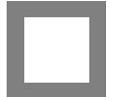Solution:
QUESTION: 17

Find the number of lines of symmetry in the below figure: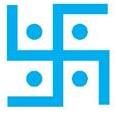Solution:
QUESTION: 18

Find the number of lines of symmetry in the below figure: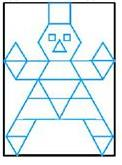Solution:
QUESTION: 19

Find the number of lines of symmetry in the below figure: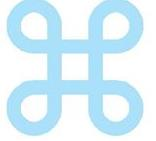Solution:
QUESTION: 20

Find the number of lines of symmetry in a circle.

Solution: